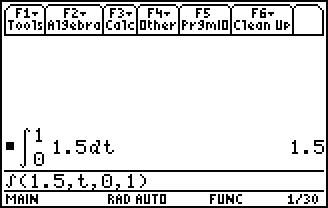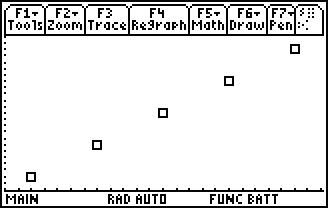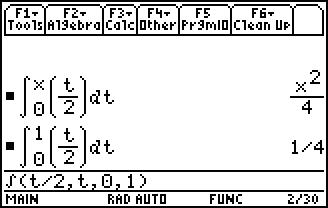# Activities

••• ##### Subject Area

• Math: Calculus: Fundamental Theorem

• ##### Author9-12

45 Minutes

• ##### Device
• TI-89 / TI-89 Titanium

## Introduction to the Fundamental Theorem#### Activity Overview

This activity builds student comprehension of functions defined by a definite integral, where the independent variable is an upper limit of integration.

#### Key Steps

•Students will compare constant and non-constant integrands.

First, students will examine a constant integrand.

•Students will notice that there is a constant rate of change, of 1.5, in the graph of f (x) = 1.5.

They will record the outputs and create a scatter plot of the ordered pairs. They will see that the graph is a line with a slope of the constant.

•In problem 2, students investigate the behavior of a non-constant integrand.

They will see that the function changes at a non-constant rate.